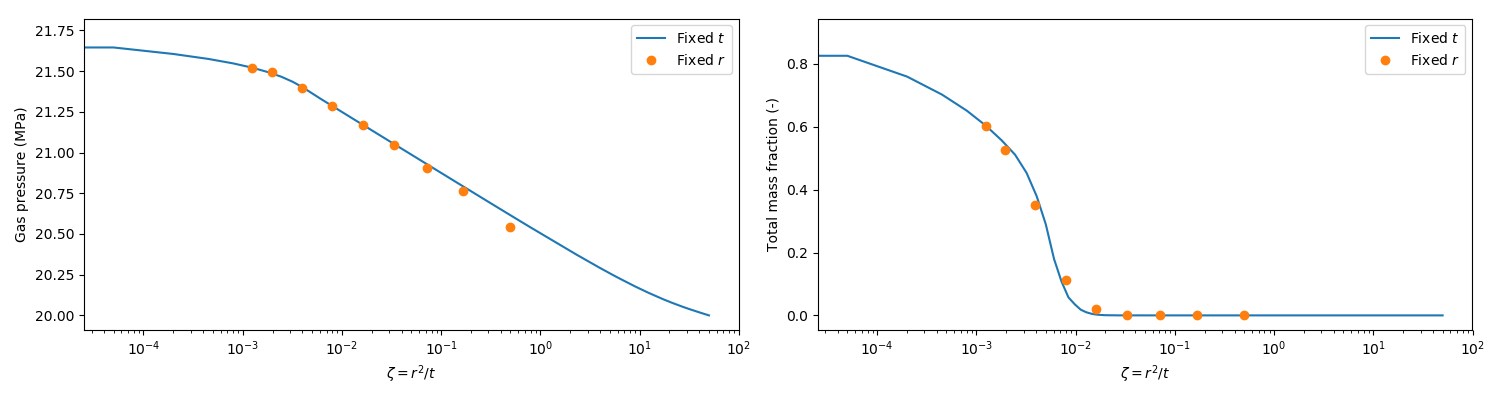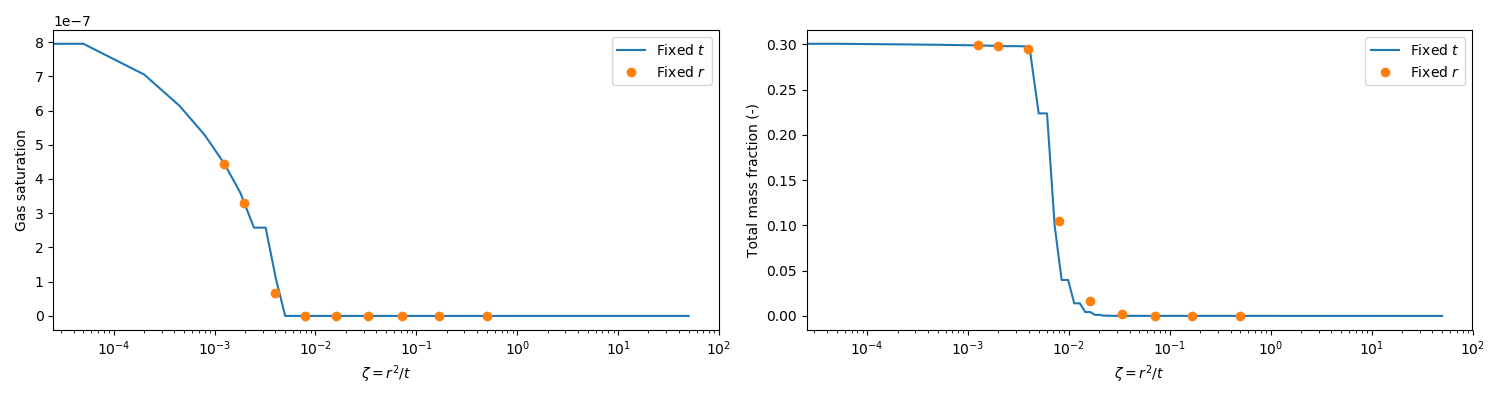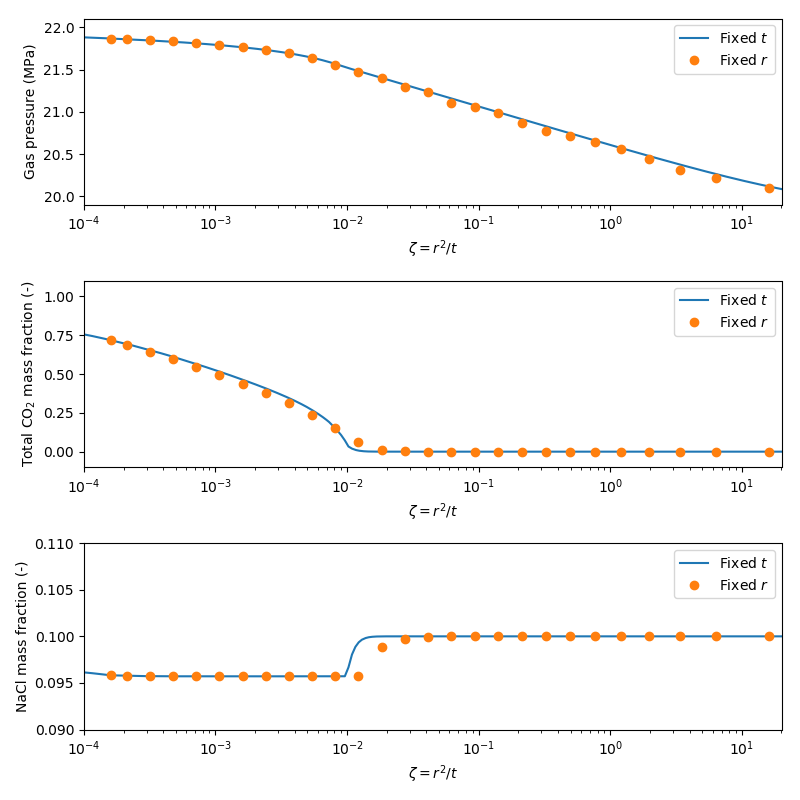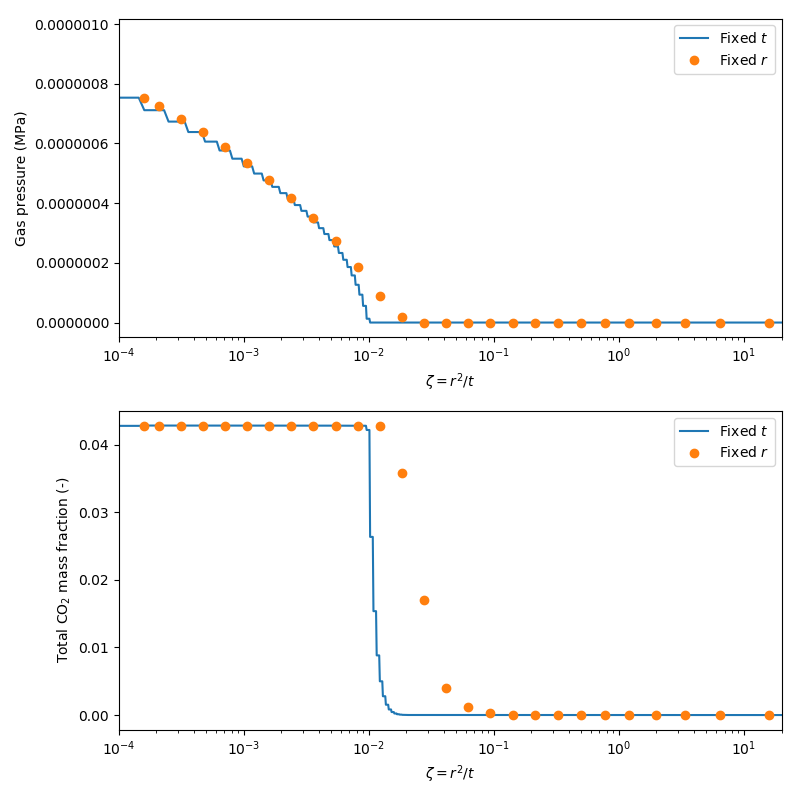# Multi-phase, multi-component radial injection

Theis pumping tests are described in the page on pumping tests and the page on point sources. This page describes similar tests, but in the multi-phase, multi-component setting.

## The similarity solution

Flow from a source in a radial 1D problem admits a similarity solution that is valid for a broad range of problems, including multi-component, multi-phase flow. Properties such as fluid pressure, saturation and component mass fraction can all be characterised by the similarity variable , where is the radial distance from the source, and is time.

This similarity solution can be used to verify simple 1D radial models of increasing complexity.

## Two phase immiscible flow

The simplest test case that features a similarity solution is injection of a gas phase into a fully liquid saturated model at a constant rate. This is described here.

## Two phase miscible flow

The similarity solution is also recovered even when including mutual dissolution of the gas and aqueous phases. In this example, CO is injected into a fully saturated reservoir at a constant rate, with the phase conditions calculated using the WaterNCG fluid state material that implements the persistent primary variable (in this case, the total mass fraction of CO summed over all phases). Initially, there is insufficient CO to form a gas phase, with all injected CO dissolving into the resident water.

# Two phase Theis problem: Flow from single source using WaterNCG fluidstate.
# Constant rate injection 2 kg/s
# 1D cylindrical mesh
# Initially, system has only a liquid phase, until enough gas is injected
# to form a gas phase, in which case the system becomes two phase.
# Note: this test is the same as theis.i, but uses the tabulated version of the CO2FluidProperties

[Mesh]
type = GeneratedMesh
dim = 1
nx = 80
xmax = 200
bias_x = 1.05
[]

[Problem]
type = FEProblem
coord_type = RZ
rz_coord_axis = Y
[]

[GlobalParams]
PorousFlowDictator = dictator
gravity = '0 0 0'
[]

[AuxVariables]
[./saturation_gas]
order = CONSTANT
family = MONOMIAL
[../]
[./x1]
order = CONSTANT
family = MONOMIAL
[../]
[./y0]
order = CONSTANT
family = MONOMIAL
[../]
[]

[AuxKernels]
[./saturation_gas]
type = PorousFlowPropertyAux
variable = saturation_gas
property = saturation
phase = 1
execute_on = timestep_end
[../]
[./x1]
type = PorousFlowPropertyAux
variable = x1
property = mass_fraction
phase = 0
fluid_component = 1
execute_on = timestep_end
[../]
[./y0]
type = PorousFlowPropertyAux
variable = y0
property = mass_fraction
phase = 1
fluid_component = 0
execute_on = timestep_end
[../]
[]

[Variables]
[./pgas]
initial_condition = 20e6
[../]
[./zi]
initial_condition = 0
[../]
[]

[Kernels]
[./mass0]
type = PorousFlowMassTimeDerivative
fluid_component = 0
variable = pgas
[../]
[./flux0]
type = PorousFlowAdvectiveFlux
fluid_component = 0
variable = pgas
[../]
[./mass1]
type = PorousFlowMassTimeDerivative
fluid_component = 1
variable = zi
[../]
[./flux1]
type = PorousFlowAdvectiveFlux
fluid_component = 1
variable = zi
[../]
[]

[UserObjects]
[./dictator]
type = PorousFlowDictator
porous_flow_vars = 'pgas zi'
number_fluid_phases = 2
number_fluid_components = 2
[../]
[./pc]
type = PorousFlowCapillaryPressureConst
pc = 0
[../]
[./fs]
type = PorousFlowWaterNCG
water_fp = water
gas_fp = tabulated
capillary_pressure = pc
[../]
[]

[Modules]
[./FluidProperties]
[./co2]
type = CO2FluidProperties
[../]
[./tabulated]
type = TabulatedFluidProperties
fp = co2
fluid_property_file = fluid_properties.csv
[../]
[./water]
type = Water97FluidProperties
[../]
[../]
[]

[Materials]
[./temperature]
type = PorousFlowTemperature
[../]
[./waterncg]
type = PorousFlowFluidState
gas_porepressure = pgas
z = zi
temperature_unit = Celsius
capillary_pressure = pc
fluid_state = fs
[../]
[./porosity]
type = PorousFlowPorosityConst
porosity = 0.2
[../]
[./permeability]
type = PorousFlowPermeabilityConst
permeability = '1e-12 0 0 0 1e-12 0 0 0 1e-12'
[../]
[./relperm_water]
type = PorousFlowRelativePermeabilityCorey
n = 2
phase = 0
s_res = 0.1
sum_s_res = 0.1
[../]
[./relperm_gas]
type = PorousFlowRelativePermeabilityCorey
n = 2
phase = 1
[../]
[]

[BCs]
[./rightwater]
type = DirichletBC
boundary = right
value = 20e6
variable = pgas
[../]
[]

[DiracKernels]
[./source]
type = PorousFlowSquarePulsePointSource
point = '0 0 0'
mass_flux = 2
variable = zi
[../]
[]

[Preconditioning]
[./smp]
type = SMP
full = true
petsc_options = '-snes_converged_reason -ksp_diagonal_scale -ksp_diagonal_scale_fix -ksp_gmres_modifiedgramschmidt -snes_linesearch_monitor'
petsc_options_iname = '-ksp_type -pc_type -sub_pc_type -sub_pc_factor_shift_type -pc_asm_overlap -snes_atol -snes_rtol -snes_max_it'
petsc_options_value = 'gmres      asm      lu           NONZERO                   2               1E-8       1E-10 20'
[../]
[]

[Executioner]
type = Transient
solve_type = NEWTON
end_time = 8e2
[./TimeStepper]
type = IterationAdaptiveDT
dt = 2
growth_factor = 2
[../]
[]

[VectorPostprocessors]
[./line]
type = LineValueSampler
sort_by = x
start_point = '0 0 0'
end_point = '200 0 0'
num_points = 1000
variable = 'pgas zi x1 saturation_gas'
execute_on = 'timestep_end'
[../]
[]

[Postprocessors]
[./pgas]
type = PointValue
point =  '1 0 0'
variable = pgas
[../]
[./sgas]
type = PointValue
point =  '1 0 0'
variable = saturation_gas
[../]
[./zi]
type = PointValue
point = '1 0 0'
variable = zi
[../]
[./massgas]
type = PorousFlowFluidMass
fluid_component = 1
[../]
[./x1]
type = PointValue
point =  '1 0 0'
variable = x1
[../]
[./y0]
type = PointValue
point =  '1 0 0'
variable = y0
[../]
[]

[Outputs]
print_linear_residuals = false
perf_graph = true
[./csvout]
type = CSV
file_base = theis_tabulated_csvout
execute_on = timestep_end
execute_vector_postprocessors_on = final
[../]
[]

(modules/porous_flow/test/tests/fluidstate/theis_tabulated.i)

After approximately 3000s, enough CO has been injected to form a gas phase, and the problem evolves from a single liquid phase to one including both gas and liquid phases. Figure 1 shows the comparison of similarity solutions calculated with either fixed radial distance or fixed time. In this case, good agreement is observed between the two results for both primary variables (gas pressure and total mass fraction of CO). The similarity solution is also observed in Figure 2 for both the calculated gas saturation and the mass fraction of CO dissolved in the water.

Since this test is part of the automatic test suite, it needs to run rapidly. Better agreement between the similarity solutions are obtained by refining the spatial and temporal resolution.Figure 1: Comparison of similarity solutions for the water-NCG fluid state. (a) Gas pressure; (b) Total mass fraction of CO2.Figure 2: Comparison of similarity solutions for the water-NCG fluid state. (a) Gas saturation; (b) Dissolved CO2 mass fraction.

## COvar element = document.getElementById("moose-equation-61fa1a16-12ba-4eda-8164-77e5184514be");katex.render("_{2}", element, {macros:{"\\pf":"\\frac{\\partial #1}{\\partial #2}"},throwOnError:false,displayMode:false}); injection into brine

Similar results are obtained for CO injection in brine, using the BrineCO2 fluid state material. In this example, CO is injected into a reservoir at a constant rate. Initially, the reservoir is fully saturated with brine which has a salt mass fraction of 0.1. During the early injection period, there is insufficient CO to form a gas phase, with all injected CO dissolving into the resident brine.

# Two phase Theis problem: Flow from single source.
# Constant rate injection 2 kg/s
# 1D cylindrical mesh
# Initially, system has only a liquid phase, until enough gas is injected
# to form a gas phase, in which case the system becomes two phase.
#
# This test takes a few minutes to run, so is marked heavy

[Mesh]
type = GeneratedMesh
dim = 1
nx = 2000
xmax = 2000
[]

[Problem]
type = FEProblem
coord_type = RZ
rz_coord_axis = Y
[]

[GlobalParams]
PorousFlowDictator = dictator
gravity = '0 0 0'
[]

[AuxVariables]
[./saturation_gas]
order = CONSTANT
family = MONOMIAL
[../]
[./x1]
order = CONSTANT
family = MONOMIAL
[../]
[./y0]
order = CONSTANT
family = MONOMIAL
[../]
[]

[AuxKernels]
[./saturation_gas]
type = PorousFlowPropertyAux
variable = saturation_gas
property = saturation
phase = 1
execute_on = timestep_end
[../]
[./x1]
type = PorousFlowPropertyAux
variable = x1
property = mass_fraction
phase = 0
fluid_component = 1
execute_on = timestep_end
[../]
[./y0]
type = PorousFlowPropertyAux
variable = y0
property = mass_fraction
phase = 1
fluid_component = 0
execute_on = timestep_end
[../]
[]

[Variables]
[./pgas]
initial_condition = 20e6
[../]
[./zi]
initial_condition = 0
[../]
[./xnacl]
initial_condition = 0.1
[../]
[]

[Kernels]
[./mass0]
type = PorousFlowMassTimeDerivative
fluid_component = 0
variable = pgas
[../]
[./flux0]
type = PorousFlowAdvectiveFlux
fluid_component = 0
variable = pgas
[../]
[./mass1]
type = PorousFlowMassTimeDerivative
fluid_component = 1
variable = zi
[../]
[./flux1]
type = PorousFlowAdvectiveFlux
fluid_component = 1
variable = zi
[../]
[./mass2]
type = PorousFlowMassTimeDerivative
fluid_component = 2
variable = xnacl
[../]
[./flux2]
type = PorousFlowAdvectiveFlux
fluid_component = 2
variable = xnacl
[../]
[]

[UserObjects]
[./dictator]
type = PorousFlowDictator
porous_flow_vars = 'pgas zi xnacl'
number_fluid_phases = 2
number_fluid_components = 3
[../]
[./pc]
type = PorousFlowCapillaryPressureConst
pc = 0
[../]
[./fs]
type = PorousFlowBrineCO2
brine_fp = brine
co2_fp = co2
capillary_pressure = pc
[../]
[]

[Modules]
[./FluidProperties]
[./co2sw]
type = CO2FluidProperties
[../]
[./co2]
type = TabulatedFluidProperties
fp = co2sw
[../]
[./water]
type = Water97FluidProperties
[../]
[./watertab]
type = TabulatedFluidProperties
fp = water
temperature_min = 273.15
temperature_max = 573.15
fluid_property_file = water_fluid_properties.csv
save_file = false
[../]
[./brine]
type = BrineFluidProperties
water_fp = watertab
[../]
[../]
[]

[Materials]
[./temperature]
type = PorousFlowTemperature
[../]
[./brineco2]
type = PorousFlowFluidState
gas_porepressure = pgas
z = zi
temperature_unit = Celsius
xnacl = xnacl
capillary_pressure = pc
fluid_state = fs
[../]
[./porosity]
type = PorousFlowPorosityConst
porosity = 0.2
[../]
[./permeability]
type = PorousFlowPermeabilityConst
permeability = '1e-12 0 0 0 1e-12 0 0 0 1e-12'
[../]
[./relperm_water]
type = PorousFlowRelativePermeabilityCorey
n = 2
phase = 0
s_res = 0.1
sum_s_res = 0.1
[../]
[./relperm_gas]
type = PorousFlowRelativePermeabilityCorey
n = 2
phase = 1
[../]
[]

[BCs]
[./rightwater]
type = DirichletBC
boundary = right
value = 20e6
variable = pgas
[../]
[]

[DiracKernels]
[./source]
type = PorousFlowSquarePulsePointSource
point = '0 0 0'
mass_flux = 2
variable = zi
[../]
[]

[Preconditioning]
[./smp]
type = SMP
full = true
[../]
[]

[Executioner]
type = Transient
solve_type = NEWTON
end_time = 1e5
[./TimeStepper]
type = IterationAdaptiveDT
dt = 1
growth_factor = 1.5
[../]
[]

[VectorPostprocessors]
[./line]
type = LineValueSampler
sort_by = x
start_point = '0 0 0'
end_point = '2000 0 0'
num_points = 10000
variable = 'pgas zi xnacl x1 saturation_gas'
execute_on = 'timestep_end'
[../]
[]

[Postprocessors]
[./pgas]
type = PointValue
point = '4 0 0'
variable = pgas
[../]
[./sgas]
type = PointValue
point = '4 0 0'
variable = saturation_gas
[../]
[./zi]
type = PointValue
point = '4 0 0'
variable = zi
[../]
[./massgas]
type = PorousFlowFluidMass
fluid_component = 1
[../]
[./x1]
type = PointValue
point = '4 0 0'
variable = x1
[../]
[./y0]
type = PointValue
point = '4 0 0'
variable = y0
[../]
[./xnacl]
type = PointValue
point = '4 0 0'
variable = xnacl
[../]
[]

[Outputs]
print_linear_residuals = false
perf_graph = true
[./csvout]
type = CSV
execute_on = timestep_end
execute_vector_postprocessors_on = final
[../]
[]

(modules/porous_flow/test/tests/fluidstate/theis_brineco2.i)

After approximately 2000s, enough CO has been injected to form a gas phase, and the problem evolves from a single liquid phase to one including both gas and liquid phases. Note that this time is earlier than the problem with water instead of brine, as the equilibrium saturation of CO in brine is less than that in water. Figure 3 shows the comparison of similarity solutions calculated with either fixed radial distance or fixed time. In this case, good agreement is observed between the two results for both primary variables (gas pressure and total mass fraction of CO). The similarity solution is also observed in Figure 4 for both the calculated gas saturation and the mass fraction of CO dissolved in the water.

This example is included in the automatic test suite, but is marked heavy due to the run time.Figure 3: Comparison of similarity solutions for the brine-CO2 fluid state. (a) Gas pressure; (b) Total mass fraction of CO2; (c) NaCl mass fraction.Figure 4: Comparison of similarity solutions for the brine-CO2 fluid state. (a) Gas saturation; (b) Dissolved CO2 mass fraction.